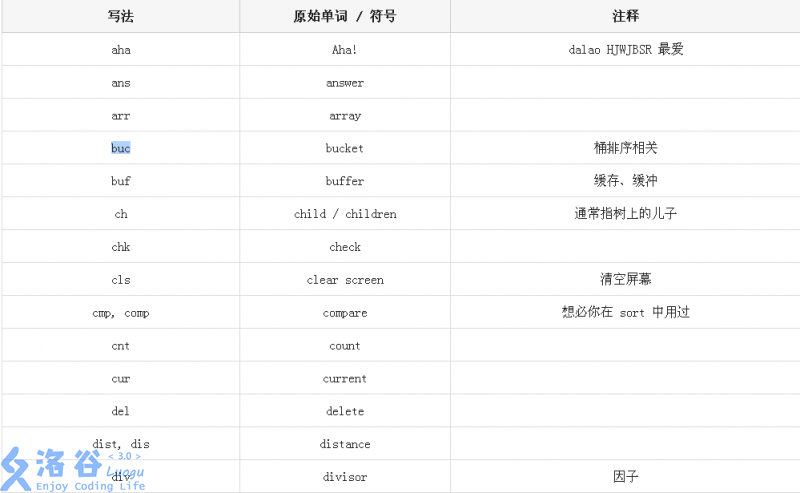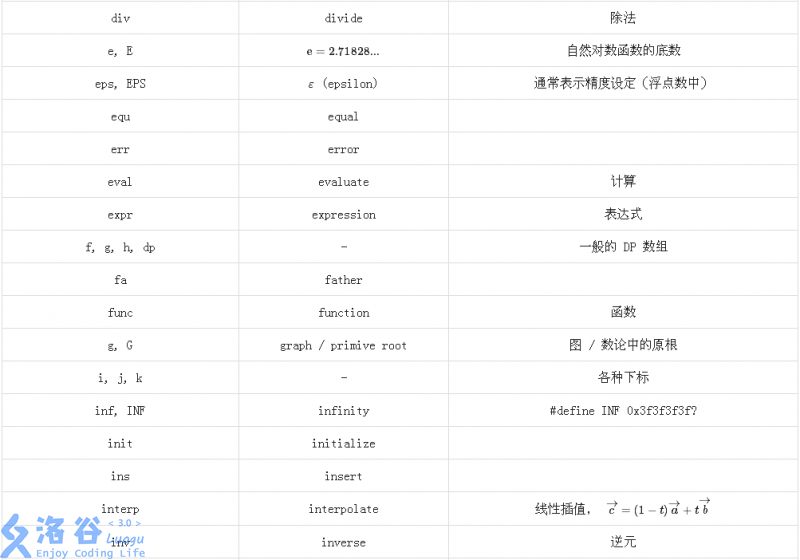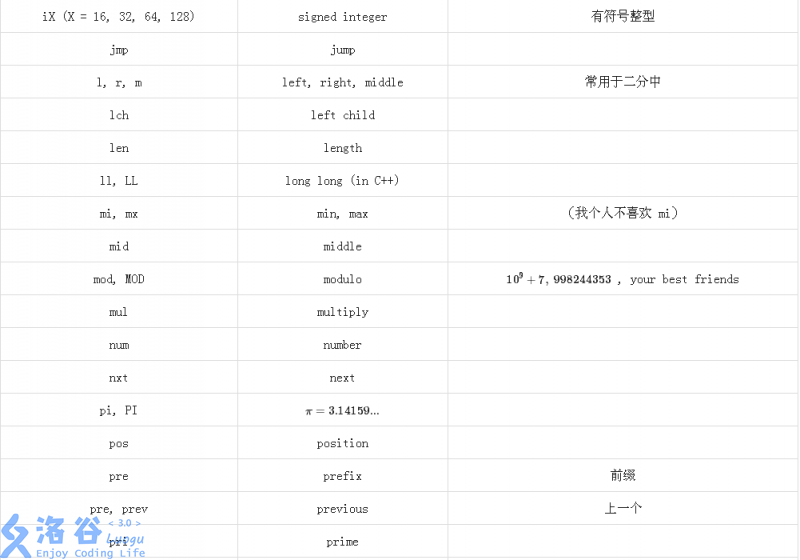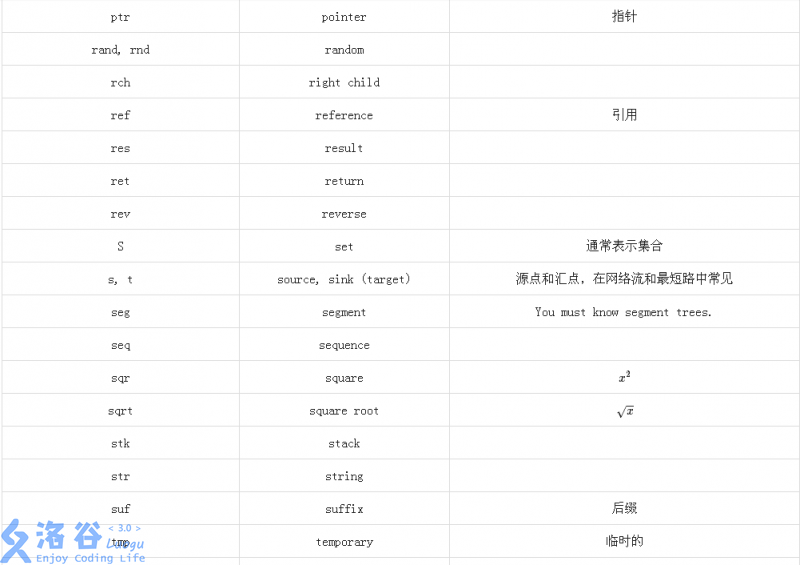## 代码结构

// 开头注释，或是一些奇怪的东西

#include <...>
#include <...>
#include <...>
// #include <bits/stdc++.h>: someone may love it. This is OK in NOI.

// 花式宏定义
// 一些简单的函数

struct XXPoint {
// 某某 struct
};

struct XXTree {
// 某某数据结构
};

void aaa() {
// ...
}

inline int bbb() {
// ...
}

// ...

int main() {
// 开文件
init();
work();
return 0;
}

## 空格与空行

x1=-b+sqrt(b*b-4*a*c)/2/a;x2=-b-sqrt(b*b-4*a*c)/(2*a);// 解方程

x1 = -b + sqrt(b * b - 4 * a * c) / 2 / a;
x2 = -b - sqrt(b * b - 4 * a * c) / (2 * a);  // 注释与代码之间通常也有 1-2 个的空格

#include<cstdio>
#include<iostream>
#include<cstring>
#include<string>
using namespace std;

const int N=1e7+1e5;

int n,m;

int prime[N],pn;
bool notprime[N];

void get_prime(){
pn=0;
notprime=true;

for(int i = 1;i <= n;i++)
{
if(!notprime[i])
{
prime[pn++]=i;
}
for(int j = 0;j < pn && prime[j] * i <= n;j++)
{
notprime[prime[j] * i]=true;
if(!(i % prime[j]))break;
}
}
}
int main()
{
int q;
scanf("%d%d",&n,&m);

get_prime();

for(int i = 1;i <= m;i++)
{
scanf("%d",&q);
if(notprime[q])
{
printf("No\n");
}else printf("Yes\n");
}

return 0;
} 

1. for循环后的花括号 尽量换行 如果有循环嵌套 不要把一堆花括号写在一起

比如

for(int i = 1;i <= n;i++)
{
for(int j = 1;j <= m;j++)
{
......
}
}//可能会好看点

再或者

    while(...){
...
}
do{

}while(...)

1. if语句

if(...)
{
....
}else if(...)
{

}else{
...
}

或者

if(...){
...
}else{
...
}

## 命名习惯